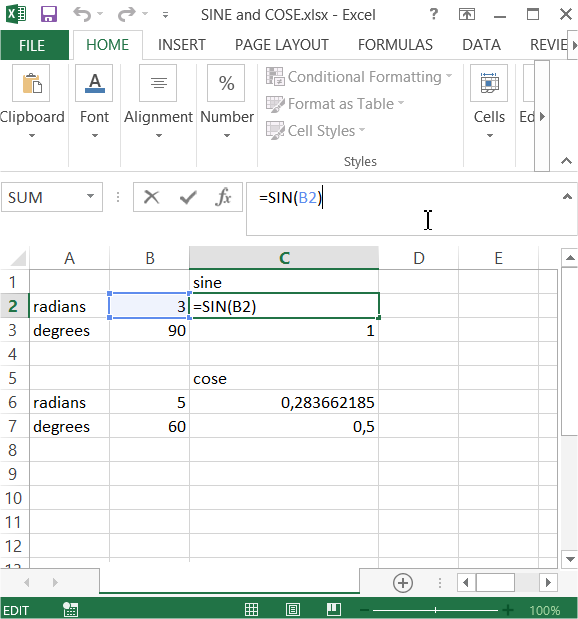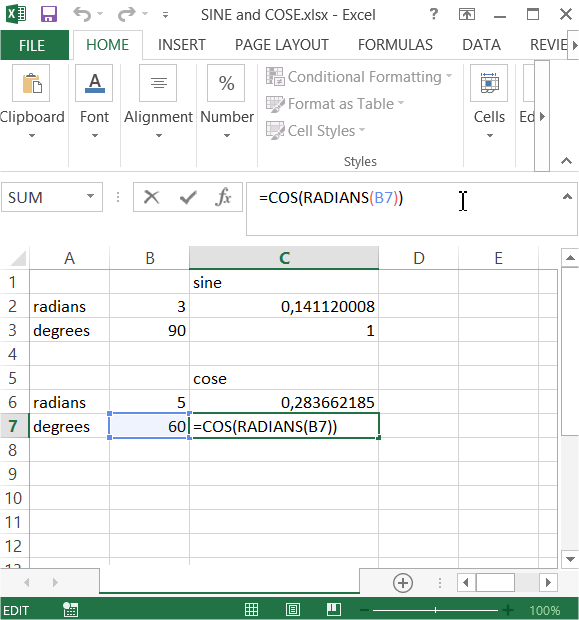## Calculating sine and cosine of an angle

In this Excel tutorial you will teach yourself how to calculate sine and cosine of an angle in a clever way. First you must realize yourself that Excel prefers radians to degrees. This is really annoying. Here you will see how to calculate sine and cosine when you have radians or degrees.To calculate sine of an angle you can just use SIN function. Formula is =SIN(radians)But what to do when you know only degrees? Excel has also RADIANS function built-in =RADIANS(degrees) which convert degrees to radians. Just use it. Formula for sine with degrees is =SIN(RADIANS(degrees))When you know only degrees just use this formula =COS(RADIANS(degrees))That’s the clever Excel trick how to convert degrees to radians and calculate sine and cosine for an angle. Trig functions are much easier thanks to Excel.

## Template

```Further reading: